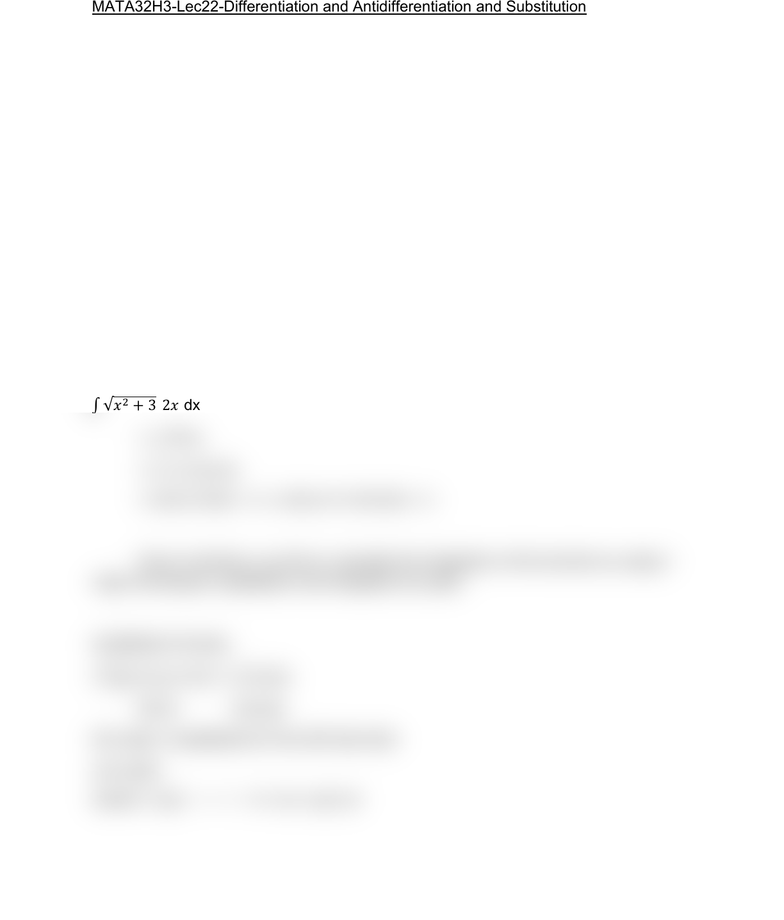Class Notes (1,100,000)
CA (640,000)
UTSC (30,000)
Mathematics (1,000)
MATA32H3 (200)
Lecture 22

# MATA32H3 Lecture Notes - Lecture 22: AntiderivativePremium

Department
Mathematics
Course Code
MATA32H3
Professor
Karimian Pour, C.
Lecture
22

This preview shows half of the first page. to view the full 2 pages of the document.MATA32H3-Lec22-Differentiation and Antidifferentiation and Substitution
Differentiation and Antidifferentiation
Differentiation and antidifferentiation are almost reverse processes.
(d/dx) (dx) = f(x)
Say f(x) = x^2 (d/dx) ( dx) = (d/dx) ((x^3 /3) + C)
= (d/dx) (x^3 /3) = x^2
Substitution
The idea of the substitution method: Given dx, depending on the special
form of g(x), we’ll perform a substitution to make it “easier”.
Example:
 dx
= 
= ) du
= (2/3) u^(3/2) + C = (2/3) (x^2+3)^(3/2) + C
Given a function, you find or calculate the integration of the function by using 2
major techniques, substitution and integration by parts.
Substitution formula:
 = 
Given Can get
We make 2 substitutions in the left hand side
Let u=g(x)
(du/dx) = g’(x) du = g’(x) dx